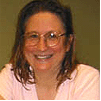top of page

# Math 4

Math 4

Saturdays, 11:10-12:25pm

Teacher: Mary Marvin

Homework Hour: Thursdays, 7:30-8:30 pm

Assistant Teacher: Timothy Torubarov

Start date: 01/07/2023

No classes on Memorial weekend. End on06/17

Total #23 weeks

Tuition is paid in two installments. The first installment is paid for classes from Jan 7th to  April 10th 2023

Curriculum

Students will move further into the topic of fractions, including adding and subtracting mixed numbers with different denominators.  Fraction multiplication will be introduced.  There will be many word problems requiring an understanding of these fraction concepts.   Long division and multiplication will now include 3, and 4-digit numbers, and prime factorization and divisibility rules will be studied.  The second semester will see the introduction of decimals and how they relate to fractions.  Geometry will be developed further, with more complex problems on area and perimeter, and now the volume of cubes and cuboids.  Along with the heavy arithmetic program, students will study exciting topics to prepare them for Math Olympiads, which they’ll start taking this year for the first time.  Topics like set theory, probability, logic, and combinatorics will be taught – if taught at an age-appropriate level, young kids can do these things.

Prerequisites

Students should have a strong mastery of arithmetic through 3rd grade to succeed in this class.  They must be able to add and subtract 4-digit numbers with “borrowing,” to multiply and divide a 3-digit by a 1-digit number, and an understanding of fractions as an equal part of the whole, along with equivalent fractions, adding and subtracting fractions with the same denominator, and basic notions of perimeter, area, and angles.

## Back to Schedule